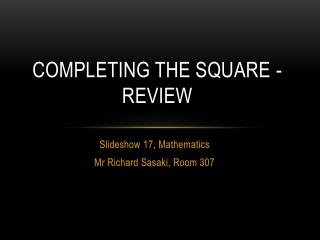DownloadDownload PresentationCompleting the Square - Review

# Completing the Square - Review

Download Presentation## Completing the Square - Review

- - - - - - - - - - - - - - - - - - - - - - - - - - - E N D - - - - - - - - - - - - - - - - - - - - - - - - - - -
##### Presentation Transcript

1. Completing the Square - Review Slideshow 17, Mathematics Mr Richard Sasaki, Room 307

2. Objectives • Recall how to complete the square (writing a quadratic equation in the form (x + a)2 = b • Solve quadratic equations through completing the square.

3. Completing the Square Let’s recall how we complete the square. Example Solve + 12 + 35 = 0. + = -35 1. Move the constant to the right. 2. Halve and square the x-coefficient. + + = 1 3. Add this to both sides. = 1 3. Factorise the left (the number is half of the x-coefficient. = ±1 3. Square root and solve. = ±1 6 = -5 or -7

4. Completing the Square Remember if the x-coefficient is negative, the symbol in the bracket is also negative. Try the worksheets, good luck. Example Solve - 12 + 27 = 0. - = -27 1. Move the constant to the right. 2. Halve and square the x-coefficient. - + = 9 3. Add this to both sides. = 9 3. Factorise the left (the number is half of the x-coefficient. = ±3 3. Square root and solve. = ±3 6 = 3 or 9

5. Answers – Top (Sheet 1) = 2 or -4 -5 or -9 3 or -1 49

6. Answers – Bottom (Sheet 1) = -4 or 6 = -7 or -1 = 11 or -5 = 25 or 3 = 7 or -9 Because adding to –b may be very difficult to square root.

7. Answers – Further Practice = -1 or 5 = -2 or 4 = 6 or 8 = -5 or 1 = -7 or 3 = -3 or 5 = -6 or 12 = -3 or 17